# Electronics and Communication Engineering - Exam Questions Papers

31.

Which one of the following statements is correct?

 A. AGC in radio receivers will keep the total signal output fairly constant but the noise component will be reduced; with the result the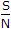ratio will improve. B. AGC in radio receivers is a linear operation w.r.t. both signals and noise C. Sudden changes in the output while tuning, which may cause damage to the components, is an unavoidable feature of AGC D. AGC operation is independent of the filter components used in AGC circuit.

Answer: Option C

Explanation:

No answer description available for this question. Let us discuss.

32.

Which of the following is not a conducting material?

 A. Copper B. Tungsten C. Germanium D. Platinum

Answer: Option C

Explanation:

No answer description available for this question. Let us discuss.

33.

The time domain behaviour of an RL circuit is represented L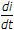+ Ri = V0 (1 + Be-Rt/L sint)u(t)
For an initial current of i(0) =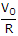, the steady state value of the current is given by

 A.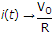B.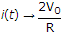C.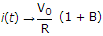D.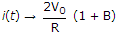Answer: Option A

Explanation:

No answer description available for this question. Let us discuss.

34.

Which of the following is true?

 A. A silicon wafer heavily doped boron is a p+ substrate B. A silicon wafer lightly doped boron is a p+ substrate C. A silicon wafer heavily doped arsenic is a p+ substrate D. A silicon wafer lightly doped arsenic is a p+ substrate

Answer: Option A

Explanation:

No answer description available for this question. Let us discuss.

35.

A standard waveguide W R90 has inside wall dimensions of a = 2.286 cm and b = 1.016 cm. What is the cut-off wavelength for TE01 mode?

 A. 4.572 cm B. 2.286 cm C. 2.032 cm D. 1.857 cm

Answer: Option C

Explanation:

No answer description available for this question. Let us discuss.

#### Current Affairs 2021

Interview Questions and Answers